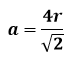# Problem: In solid KCl the smallest distance between the centers of a potassium ion and a chloride ion is 314 pm. Calculate the length of the edge of the unit cell and the density of KCl, assuming it has the same structure as sodium chloride.

###### FREE Expert Solution

Sodium chloride is face-centered cubic unit cell. Recall that a Face-Centered Cubic (FCC) unit cell has 4 atoms per unit cell.

And the formula for the edge length (a) of FCC unit cell:Since the given is the distance between the center of potassium ion and chloride ion (not only the radius of one ion), the equation becomes:

88% (442 ratings)###### Problem Details

In solid KCl the smallest distance between the centers of a potassium ion and a chloride ion is 314 pm. Calculate the length of the edge of the unit cell and the density of KCl, assuming it has the same structure as sodium chloride.